# Catenary

"Chainette" redirects here. For the wine grape also known as Chainette, see Cinsaut.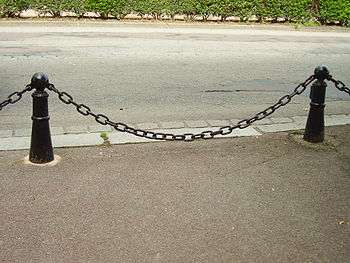A chain hanging from points forms a catenary.Freely-hanging electric power cables (especially those used on electrified railways) can also form a catenary.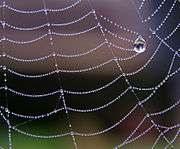The silk on a spider's web forming multiple elastic catenaries.

In physics and geometry, a catenary (US /ˈkætənˌɛri/, UK /kəˈtnəri/) is the curve that an idealized hanging chain or cable assumes under its own weight when supported only at its ends. The curve has a U-like shape, superficially similar in appearance to a parabola, but it is not a parabola: it is a (scaled, rotated) graph of the hyperbolic cosine. The curve appears in the design of certain types of arches and as a cross section of the catenoid—the shape assumed by a soap film bounded by two parallel circular rings.

The catenary is also called the alysoid, chainette, or, particularly in the materials sciences, funicular.

Mathematically, the catenary curve is the graph of the hyperbolic cosine function. The surface of revolution of the catenary curve, the catenoid, is a minimal surface, specifically a minimal surface of revolution. The mathematical properties of the catenary curve were first studied by Robert Hooke in the 1670s, and its equation was derived by Leibniz, Huygens and Johann Bernoulli in 1691.

Catenaries and related curves are used in architecture and engineering, in the design of bridges and arches, so that forces do not result in bending moments. In the offshore oil and gas industry, "catenary" refers to a steel catenary riser, a pipeline suspended between a production platform and the seabed that adopts an approximate catenary shape.

## History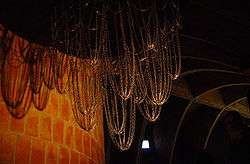Antoni Gaudí's catenary model at Casa Milà

The word "catenary" is derived from the Latin word catēna, which means "chain". The English word "catenary" is usually attributed to Thomas Jefferson, who wrote in a letter to Thomas Paine on the construction of an arch for a bridge:

I have lately received from Italy a treatise on the equilibrium of arches, by the Abbé Mascheroni. It appears to be a very scientifical work. I have not yet had time to engage in it; but I find that the conclusions of his demonstrations are, that every part of the catenary is in perfect equilibrium.


It is often said that Galileo thought the curve of a hanging chain was parabolic. In his Two New Sciences (1638), Galileo says that a hanging cord is an approximate parabola, and he correctly observes that this approximation improves as the curvature gets smaller and is almost exact when the elevation is less than 45°. That the curve followed by a chain is not a parabola was proven by Joachim Jungius (1587–1657); this result was published posthumously in 1669.

The application of the catenary to the construction of arches is attributed to Robert Hooke, whose "true mathematical and mechanical form" in the context of the rebuilding of St Paul's Cathedral alluded to a catenary. Some much older arches approximate catenaries, an example of which is the Arch of Taq-i Kisra in Ctesiphon.

In 1671, Hooke announced to the Royal Society that he had solved the problem of the optimal shape of an arch, and in 1675 published an encrypted solution as a Latin anagram in an appendix to his Description of Helioscopes, where he wrote that he had found "a true mathematical and mechanical form of all manner of Arches for Building." He did not publish the solution to this anagram in his lifetime, but in 1705 his executor provided it as ut pendet continuum flexile, sic stabit contiguum rigidum inversum, meaning "As hangs a flexible cable so, inverted, stand the touching pieces of an arch."

In 1691, Gottfried Leibniz, Christiaan Huygens, and Johann Bernoulli derived the equation in response to a challenge by Jakob Bernoulli; their solutions were published in the Acta Eruditorum for June 1691. David Gregory wrote a treatise on the catenary in 1697 in which he provided an incorrect derivation of the correct differential equation.

Euler proved in 1744 that the catenary is the curve which, when rotated about the x-axis, gives the surface of minimum surface area (the catenoid) for the given bounding circles. Nicolas Fuss gave equations describing the equilibrium of a chain under any force in 1796.

## Inverted catenary arch

Catenary arches are often used in the construction of kilns. To create the desired curve, the shape of a hanging chain of the desired dimensions is transferred to a form which is then used as a guide for the placement of bricks or other building material.

The Gateway Arch in St. Louis, Missouri, United States is sometimes said to be an (inverted) catenary, but this is incorrect. It is close to a more general curve called a flattened catenary, with equation y = A cosh(Bx), which is a catenary if AB = 1. While a catenary is the ideal shape for a freestanding arch of constant thickness, the Gateway Arch is narrower near the top. According to the U.S. National Historic Landmark nomination for the arch, it is a "weighted catenary" instead. Its shape corresponds to the shape that a weighted chain, having lighter links in the middle, would form.

Inverted catenary arches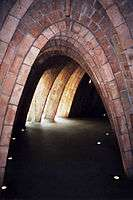Catenary arches under the roof of Gaudí's Casa Milà, Barcelona, Spain.The Sheffield Winter Garden is enclosed by a series of catenary arches.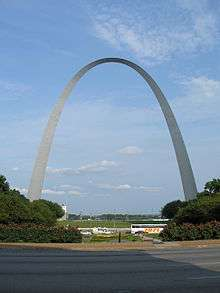The Gateway Arch (looking East) is a flattened catenary.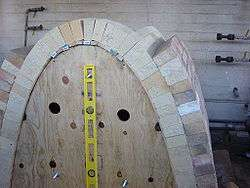Catenary arch kiln under construction over temporary formCross-section of the roof the Keleti Railway Station (Budapest, Hungary).Cross-section of the roof the Keleti Railway Station forms a catenary.

## Catenary bridges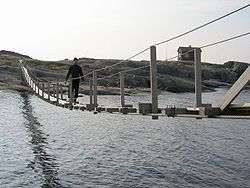Simple suspension bridges are essentially thickened cables, and follow a catenary curve.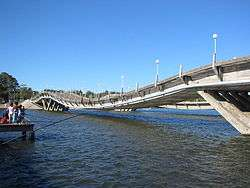Stressed ribbon bridges, like this one in Maldonado, Uruguay, also follow a catenary curve, with cables embedded in a rigid deck.

In free-hanging chains, the force exerted is uniform with respect to length of the chain, and so the chain follows the catenary curve. The same is true of a simple suspension bridge or "catenary bridge," where the roadway follows the cable.

A stressed ribbon bridge is a more sophisticated structure with the same catenary shape.

However, in a suspension bridge with a suspended roadway, the chains or cables support the weight of the bridge, and so do not hang freely. In most cases the roadway is flat, so when the weight of the cable is negligible compared with the weight being supported, the force exerted is uniform with respect to horizontal distance, and the result is a parabola, as discussed below (although the term "catenary" is often still used, in an informal sense). If the cable is heavy then the resulting curve is between a catenary and a parabola.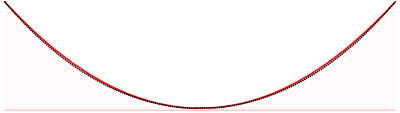Comparison of a catenary arch (black dotted curve) and a parabolic arch (red solid curve) with the same span and sag. The catenary represents the profile of a simple suspension bridge, or the cable of a suspended-deck suspension bridge on which its deck and hangers have negligible mass compared to its cable. The parabola represents the profile of the cable of a suspended-deck suspension bridge on which its cable and hangers have negligible mass compared to its deck. The profile of the cable of a real suspension bridge with the same span and sag lies between the two curves. The catenary and parabola equations are y = cosh(x) and y = (cosh(1) − 1)x2 + 1, respectively.

## Anchoring of marine objects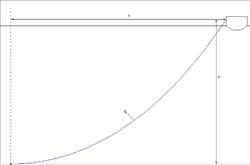A heavy anchor chain forms a catenary, with a low angle of pull on the anchor.

The catenary produced by gravity provides an advantage to heavy anchor rodes. An anchor rode (or anchor line) usually consists of chain or cable or both. Anchor rodes are used by ships, oilrigs, docks, floating wind turbines, and other marine equipment which must be anchored to the seabed.

When the rode is slack, the catenary curve presents a lower angle of pull on the anchor or mooring device than would be the case if it were nearly straight. This enhances the performance of the anchor and raises the level of force it will resist before dragging. To maintain the catenary shape in the presence of wind, a heavy chain is needed, so that only larger ships in deeper water can rely on this effect. Smaller boats must rely on the performance of the anchor itself.

## Mathematical description

### Equation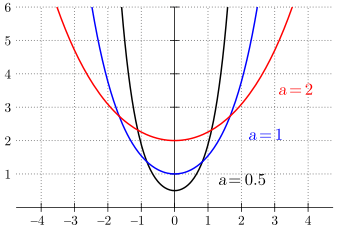Catenaries for different values of a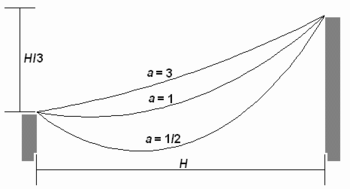Three different catenaries through the same two points, depending horizontal force TH, being a = λH/TH and λ mass per unit length.

The equation of a catenary in Cartesian coordinates has the formwhere cosh is the hyperbolic cosine function. All catenary curves are similar to each other; changing the parameter a is equivalent to a uniform scaling of the curve.

The Whewell equation for the catenary isDifferentiating givesand eliminating φ gives the Cesàro equationThe radius of curvature is thenwhich is the length of the line normal to the curve between it and the x-axis.

### Relation to other curves

When a parabola is rolled along a straight line, the roulette curve traced by its focus is a catenary. The envelope of the directrix of the parabola is also a catenary. The involute from the vertex, that is the roulette formed traced by a point starting at the vertex when a line is rolled on a catenary, is the tractrix.

Another roulette, formed by rolling a line on a catenary, is another line. This implies that square wheels can roll perfectly smoothly if the road has evenly spaced bumps in the shape of a series of inverted catenary curves. The wheels can be any regular polygon except a triangle, but the catenary must have parameters corresponding to the shape and dimensions of the wheels.

### Geometrical properties

Over any horizontal interval, the ratio of the area under the catenary to its length equals a, independent of the interval selected. The catenary is the only plane curve other than a horizontal line with this property. Also, the geometric centroid of the area under a stretch of catenary is the midpoint of the perpendicular segment connecting the centroid of the curve itself and the x-axis.

### Science

A moving charge in a uniform electric field travels along a catenary (which tends to a parabola if the charge velocity is much less than the speed of light c).

The surface of revolution with fixed radii at either end that has minimum surface area is a catenary revolved about the x-axis.

## Analysis

### Model of chains and arches

In the mathematical model the chain (or cord, cable, rope, string, etc.) is idealized by assuming that it is so thin that it can be regarded as a curve and that it is so flexible any force of tension exerted by the chain is parallel to the chain. The analysis of the curve for an optimal arch is similar except that the forces of tension become forces of compression and everything is inverted. An underlying principle is that the chain may be considered a rigid body once it has attained equilibrium. Equations which define the shape of the curve and the tension of the chain at each point may be derived by a careful inspection of the various forces acting on a segment using the fact that these forces must be in balance if the chain is in static equilibrium.

Let the path followed by the chain be given parametrically by r = (x, y) = (x(s), y(s)) where s represents arc length and r is the position vector. This is the natural parameterization and has the property thatwhere u is a unit tangent vector.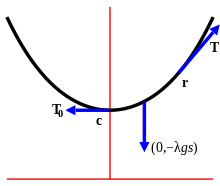Diagram of forces acting on a segment of a catenary from c to r. The forces are the tension T0 at c, the tension T at r, and the weight of the chain (0, −λgs). Since the chain is at rest the sum of these forces must be zero.

A differential equation for the curve may be derived as follows. Let c be the lowest point on the chain, called the vertex of the catenary. Assume r is to the right of c since the other case is implied by symmetry. The forces acting on the section of the chain from c to r are the tension of the chain at c, the tension of the chain at r, and the weight of the chain. The tension at c is tangent to the curve at c and is therefore horizontal without any vertical component and it pulls the section to the left so it may be written (−T0, 0) where T0 is the magnitude of the force. The tension at r is parallel to the curve at r and pulls the section to the right. The tension at r can be split into two components so it may be written Tu = (T cos φ, T sin φ), where T is the magnitude of the force and φ is the angle between the curve at r and the x-axis (see tangential angle). Finally, the weight of the chain is represented by (0, −λgs) where λ is the mass per unit length, g is the acceleration of gravity and s is the length of the segment of chain between c and r.

The chain is in equilibrium so the sum of three forces is 0, thereforeandand dividing these givesIt is convenient to writewhich is the length of chain whose weight is equal in magnitude to the tension at c. Thenis an equation defining the curve.

The horizontal component of the tension, T cos φ = T0 is constant and the vertical component of the tension, T sin φ = λgs is proportional to the length of chain between the r and the vertex.

### Derivation of equations for the curve

The differential equation given above can be solved to produce equations for the curve.

Fromthe formula for arc length givesThenandThe second of these equations can be integrated to giveand by shifting the position of the x-axis, β can be taken to be 0. ThenThe x-axis thus chosen is called the directrix of the catenary.

It follows that the magnitude of the tension at a point (x, y) is T = λgy, which is proportional to the distance between the point and the directrix.

The integral of the expression for dx/ds can be found using standard techniques, givingand, again, by shifting the position of the y-axis, α can be taken to be 0. ThenThe y-axis thus chosen passes through the vertex and is called the axis of the catenary.

These results can be used to eliminate s giving### Alternative derivation

The differential equation can be solved using a different approach. Fromit follows thatandIntegrating gives,andAs before, the x and y-axes can be shifted so α and β can be taken to be 0. Thenand taking the reciprocal of both sidesAdding and subtracting the last two equations then gives the solutionand### Determining parameters

In general the parameter a and the position of the axis. The equation can be determined in this case as follows: Relabel if necessary so that P1 is to the left of P2 and let h be the horizontal and v be the vertical distance from P1 to P2. Translate the axes so that the vertex of the catenary lies on the y-axis and its height a is adjusted so the catenary satisfies the standard equation of the curveand let the coordinates of P1 and P2 be (x1, y1) and (x2, y2) respectively. The curve passes through these points, so the difference of height isand the length of the curve from P1 to P2 isWhen s2v2 is expanded using these expressions the result issoThis is a transcendental equation in a and must be solved numerically. It can be shown with the methods of calculus that there is at most one solution with a > 0 and so there is at most one position of equilibrium.

## Generalizations with vertical force

### Nonuniform chains

If the density of the chain is variable then the analysis above can be adapted to produce equations for the curve given the density, or given the curve to find the density.

Let w denote the weight per unit length of the chain, then the weight of the chain has magnitudewhere the limits of integration are c and r. Balancing forces as in the uniform chain producesandand thereforeDifferentiation then givesIn terms of φ and the radius of curvature ρ this becomes### Suspension bridge curve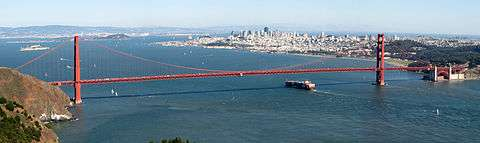Golden Gate Bridge. Most suspension bridge cables follow a parabolic, not a catenary curve, due to the weight of the roadway being much greater than that of the cable.

A similar analysis can be done to find the curve followed by the cable supporting a suspension bridge with a horizontal roadway. If the weight of the roadway per unit length is w and the weight of the cable and the wire supporting the bridge is negligible in comparison, then the weight on the cable from c to r is wx where x is the horizontal distance between c and r. Proceeding as before gives the differential equationThis is solved by simple integration to getand so the cable follows a parabola. If the weight of the cable and supporting wires are not negligible then the analysis is more complex.

### Catenary of equal strength

In a catenary of equal strength, cable is strengthened according to the magnitude of the tension at each point, so its resistance to breaking is constant along its length. Assuming that the strength of the cable is proportional to its density per unit length, the weight, w, per unit length of the chain can be written T/c, where c is constant, and the analysis for nonuniform chains can be applied.

In this case the equations for tension areCombining givesand by differentiationwhere ρ is the radius of curvature.

The solution to this isIn this case, the curve has vertical asymptotes and this limits the span to πc. Other relations areThe curve was studied 1826 by Davies Gilbert and, apparently independently, by Gaspard-Gustave Coriolis in 1836.

Recently, it was shown that this type of catenary could act as a building block of electromagnetic metasurface and was known as "catenary of equal phase gradient".

### Elastic catenary

In an elastic catenary, the chain is replaced by a spring which can stretch in response to tension. The spring is assumed to stretch in accordance with Hooke's Law. Specifically, if p is the natural length of a section of spring, then the length of the spring with tension T applied has lengthwhere E is a constant equal to kp, where k is the stiffness of the spring. In the catenary the value of T is variable, but ratio remains valid at a local level, soThe curve followed by an elastic spring can now be derived following a similar method as for the inelastic spring.

The equations for tension of the spring areandfrom whichwhere p is the natural length of the segment from c to r and λ0 is the mass per unit length of the spring with no tension and g is the acceleration of gravity. WritesoThenandfrom whichandIntegrating gives the parametric equationsAgain, the x and y-axes can be shifted so α and β can be taken to be 0. Soare parametric equations for the curve. At the rigid limit where E is large, the shape of the curve reduces to that of a non-elastic chain.

## Other generalizations

### Chain under a general force

With no assumptions have been made regarding the force G acting on the chain, the following analysis can be made.

First, let T = T(s) be the force of tension as a function of s. The chain is flexible so it can only exert a force parallel to itself. Since tension is defined as the force that the chain exerts on itself, T must be parallel to the chain. In other words,where T is the magnitude of T and u is the unit tangent vector.

Second, let G = G(s) be the external force per unit length acting on a small segment of a chain as a function of s. The forces acting on the segment of the chain between s and s + Δs are the force of tension T(s + Δs) at one end of the segment, the nearly opposite force T(s) at the other end, and the external force acting on the segment which is approximately GΔs. These forces must balance soDivide by Δs and take the limit as Δs → 0 to obtainThese equations can be used as the starting point in the analysis of a flexible chain acting under any external force. In the case of the standard catenary, G = (0, −λg) where the chain has mass λ per unit length and g is the acceleration of gravity.

1. MathWorld
2. e.g.: Shodek, Daniel L. (2004). Structures (5th ed.). Prentice Hall. p. 22. ISBN 978-0-13-048879-4. OCLC 148137330.
3. ""Catenary" at Math Words". Pballew.net. 1995-11-21. Retrieved 2010-11-17.
4. Barrow, John D. (2010). 100 Essential Things You Didn't Know You Didn't Know: Math Explains Your World. W. W. Norton & Company. p. 27. ISBN 0-393-33867-3.
5. Jefferson, Thomas (1829). Memoirs, Correspondence and Private Papers of Thomas Jefferson. Henry Colbura and Richard Bertley. p. 419.
6. Lockwood p. 124
7. Fahie, John Joseph (1903). Galileo, His Life and Work. J. Murray. pp. 359–360.
8. Jardine, Lisa. Monuments and Microscopes: Scientific Thinking on a Grand Scale in the Early Royal Society. JSTOR 532102.
9. Denny, Mark (2010). Super Structures: The Science of Bridges, Buildings, Dams, and Other Feats of Engineering. JHU Press. pp. 112–113. ISBN 0-8018-9437-9.
10. cf. the anagram for Hooke's law, which appeared in the next paragraph.
11. "Arch Design". Lindahall.org. 2002-10-28. Retrieved 2010-11-17.
12. The original anagram was abcccddeeeeefggiiiiiiiillmmmmnnnnnooprrsssttttttuuuuuuuux: the letters of the Latin phrase, alphabetized.
13. Truesdell, C. (1960), The Rotational Mechanics of Flexible Or Elastic Bodies 1638–1788: Introduction to Leonhardi Euleri Opera Omnia Vol. X et XI Seriei Secundae, Zürich: Orell Füssli, p. 66
14. Calladine, C. R. (2015-04-13), "An amateur's contribution to the design of Telford's Menai Suspension Bridge: a commentary on Gilbert (1826) 'On the mathematical theory of suspension bridges'", Philosophical Transactions of the Royal Society A, 373 (2039): 20140346, doi:10.1098/rsta.2014.0346
15. Gregorii, Davidis (August 1697), "Catenaria", Philosophical Transactions, 19 (231): 637–652, doi:10.1098/rstl.1695.0114
16. Routh Art. 455, footnote
17. Minogue, Coll; Sanderson, Robert (2000). Wood-fired Ceramics: Contemporary Practices. University of Pennsylvania. p. 42. ISBN 0-8122-3514-2.
18. Peterson, Susan; Peterson, Jan (2003). The Craft and Art of Clay: A Complete Potter's Handbook. Laurence King. p. 224. ISBN 1-85669-354-6.
19. Osserman, Robert (2010), "Mathematics of the Gateway Arch", Notices of the American Mathematical Society, 57 (2): 220–229, ISSN 0002-9920
20. Hicks, Clifford B. (December 1963). "The Incredible Gateway Arch: America's Mightiest National Monument". Popular Mechanics. Hearst Magazines. 120 (6): 89. ISSN 0032-4558.
21. Harrison, Laura Soullière (1985), National Register of Historic Places Inventory-Nomination: Jefferson National Expansion Memorial Gateway Arch / Gateway Arch; or "The Arch" (PDF), National Park Service and Accompanying one photo, aerial, from 1975 (578 KB)
22. Sennott, Stephen (2004). Encyclopedia of Twentieth Century Architecture. Taylor & Francis. p. 224. ISBN 1-57958-433-0.
23. Hymers, Paul (2005). Planning and Building a Conservatory. New Holland. p. 36. ISBN 1-84330-910-6.
24. Byer, Owen; Lazebnik, Felix; Smeltzer, Deirdre L. (2010-09-02). Methods for Euclidean Geometry. MAA. p. 210. ISBN 978-0-88385-763-2.
25. Fernández Troyano, Leonardo (2003). Bridge Engineering: A Global Perspective. Thomas Telford. p. 514. ISBN 978-0-7277-3215-6.
26. Trinks, W.; Mawhinney, M. H.; Shannon, R. A.; Reed, R. J.; Garvey, J. R. (2003-12-05). Industrial Furnaces. Wiley. p. 132. ISBN 978-0-471-38706-0.
27. Scott, John S. (1992-10-31). Dictionary Of Civil Engineering. Springer. p. 433. ISBN 978-0-412-98421-1.
28. The Architects' Journal. The Architectural Press. 207: 51. 1998. Missing or empty |title= (help)
29. Lockwood p. 122
30. Kunkel, Paul (June 30, 2006). "Hanging With Galileo". Whistler Alley Mathematics. Retrieved March 27, 2009.
31. "Chain, Rope, and Catenary – Anchor Systems For Small Boats". Petersmith.net.nz. Retrieved 2010-11-17.
32. "Catenary". Xahlee.org. 2003-05-28. Retrieved 2010-11-17.
33. MathWorld, eq. 7
34. Routh Art. 444
35. Yates, Robert C. (1952). Curves and their Properties. NCTM. p. 13.
36. Yates p. 80
37. Hall, Leon; Wagon, Stan (1992). "Roads and Wheels". Mathematics Magazine. MAA. 65 (5): 283–301. doi:10.2307/2691240.
38. Parker, Edward (2010). "A Property Characterizing the Catenary". Mathematics Magazine. 83: 63–64.
39. Landau, Lev Davidovich (1975). The Classical Theory of Fields. Butterworth-Heinemann. p. 56. ISBN 0-7506-2768-9.
40. Routh Art. 442, p. 316
41. Church, Irving Porter (1890). Mechanics of Engineering. Wiley. p. 387.
42. Whewell p. 65
43. Following Routh Art. 443 p. 316
44. Routh Art. 443 p. 317
45. Whewell p. 67
46. Routh Art. 443 p. 318
47. Following Routh Art. 443 p/ 317
48. Use of hyperbolic functions follows Maurer p. 107
49. Following Lamb p. 342
50. Following Todhunter Art. 186
51. See Routh art. 447
52. Following Routh Art. 450
53. Following Routh Art. 452
54. Ira Freeman investigated the case where only the cable and roadway are significant, see the External links section. Routh gives the case where only the supporting wires have significant weight as an exercise.
55. Following Routh Art. 453
56. Pu, Mingbo; Li, Xiong; Ma, Xiaoliang; Luo, Xiangang (2015). "Catenary Optics for Achromatic Generation of Perfect Optical Angular Momentum". Science Advances. AAAS. 1 (9): e1500396. doi:10.1126/sciadv.1500396.
57. Routh Art. 489
58. Routh Art. 494
59. Following Routh Art. 500
60. Follows Routh Art. 455

## BibliographyWikimedia Commons has media related to Catenary.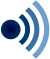Wikiquote has quotations related to: CatenaryWikisource has the text of the 1911 Encyclopædia Britannica article Catenary.